Home News About Me Ph. D. Thesis Abstract Table of Contents 1. Introduction 2. Theory  Fundamentals of the Multivariate Data Analysis 3. Theory  Quantification of the Refrigerants R22 and R134a: Part I 4. Experiments, Setups and Data Sets 5. Results  Kinetic Measurements 6. Results  Multivariate Calibrations 7. Results  Genetic Algorithm Framework 7.1. Single Run Genetic Algorithm 7.2. Genetic Algorithm Framework - Theory 7.3. Genetic Algorithm Framework - Results 7.4. Genetic Algorithm Framework  Conclusions 8. Results  Growing Neural Network Framework 9. Results  All Data Sets 10. Results  Various Aspects of the Frameworks and Measurements 11. Summary and Outlook 12. References 13. Acknowledgements Publications Research Tutorials Downloads and Links Contact Search Site Map Print this Page## 7.3.   Genetic Algorithm Framework - Results

The genetic algorithm framework was applied to the calibration data of the refrigerant data set. Thereby 100 parallel runs of the GA were used with the same settings of the parameters as described in section 7.1. The results of the first step of the global algorithm are shown in figure 46. Thereby the ranking of the variables is shown as frequency of the variables being present in the last population of the genetic algorithms. In the second step, these variables enter the model according to their rank until the prediction of the test data of a 20-fold random subsampling does not improve any more. The iterative procedure stopped after the addition of 10 time points, which are labeled in figure 46. As the labels are rounded seconds of the time points, the most important time point "0" does not represent the absolute beginning of the measurement, but 0.3 seconds after the beginning of exposure to analyte.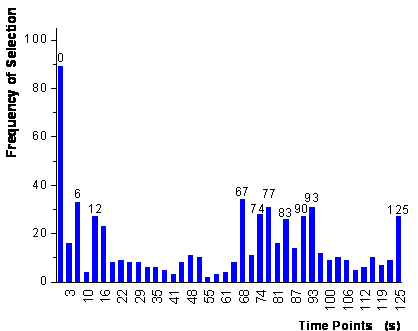figure 46:  Frequency of the selection of the time points for 100 parallel runs of the genetic algorithms. The 10 time points selected by the algorithm are labeled additionally.

The optimized networks (10 input neurons, 8 hidden neurons, 1 output neuron, fully connected) predicted the test data of the 20-fold random subsampling procedure (figure 45) with rather low rel. RMSE of 1.94% (R22) and 3.05% (R134a). The predictions of the external validation data by these networks, which had been trained by the complete calibration data set, were best of all methods used so far with 2.04% for R22 and 2.89% for R134a (see table 3 in section 7.4). Practically no gap between the prediction of the calibration and validation data is noticeable indicating much more stable models compared with the non-optimized networks. The predictions of the validation data, which are shown in the true-predicted plots in figure 47, are not biased and hardly scattered.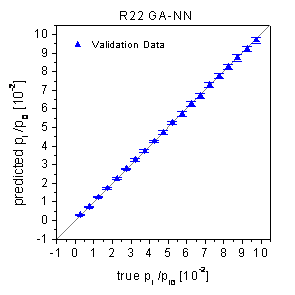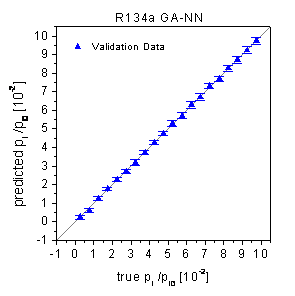figure 47:  Predictions of the validation data by neural networks optimized by the genetic algorithm framework.

The residuals of the predictions of the neural networks were further examined in respect to the compositions of the predicted analyte concentrations. In figure 48, the absolute residuals of the predictions of the analyte concentrations are plotted versus the corresponding analyte concentrations of the predicted sample. The plot for R22 demonstrates that the absolute residual for the prediction of R22 increases with an increasing concentration of R22, but is practically randomly distributed along the axis representing the concentration of R134a. For R134a the plot shows that the residuals of the predictions of R134 show a higher dependency on the concentration of R134a than on the concentration of R22. This means that the concentration of the interfering analyte does practically not influence the prediction quality of the analyte of interest. Thus, it should be possible that the system of the time-resolved measurements, the variable selection and the calibration by neural networks can be extended to parallel quantifications of even more analytes.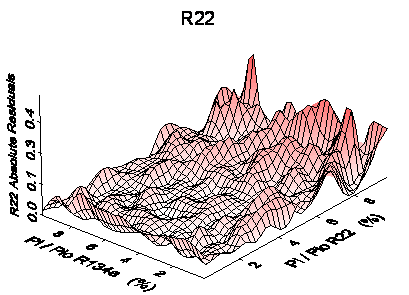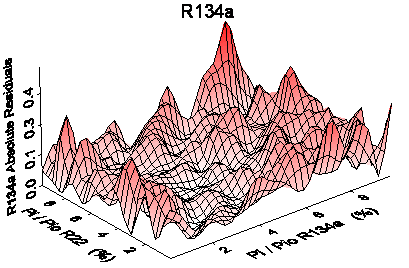figure 48:  Absolute residuals of the predictions of the concentrations of R22 (top) and R134a (bottom) versus the compositions of the corresponding samples.

An unattended use of many genetic algorithms is often limited by chance correlations of variables. This can happen if variables are noisy, if the number of samples is limited and if there are many variables to select. In that case, it can happen that the GA models noise instead of information and consequently selects randomly correlated variables. Therefore, a test similar to , was performed to investigate the robustness of the variable selection algorithm proposed in this study. In this test, the number of variables is increased by adding meaningless artificial variables, which contain only random numbers, to the meaningful original variables. Then, the algorithm for the variable selection is run using the increased amount of variables. A well performing algorithm should not select any of the artificial random variables, which contain no meaningful information. For this study, 40 random variables were added to the set of 40 original time points. The random variables were created by uniformly distributed random numbers with the same variation as the original time points. The genetic algorithm framework was used for this extended data set same way as described before except of two parameters adapted for the increased data set: The population size was increased to 100 resulting in about 120 generations until the convergence criterion was reached and the parameter a was set to 1, which resulted in approximately 6 variables being selected in single runs of the GA.

The variable ranking after the first step of the algorithm is shown in figure 49. It is obvious that all random time points are ranked very low and no random variable can be found among the most important 18 time points. The parallel runs of multiple GA with different combina­tions of test and calibration data seem to prevent the selection of randomly correlated variables whereas single runs of the GA selected random variables evident by non-zero frequencies of random variables in figure 49. Additionally, the left side of figure 49 looks very similar to figure 46 demonstrating the reproducibility of the ranking of meaningful variables when running the global algorithm repeatedly.  The top 11 time-points are ranked the same way as for the algorithm applied to the original data (figure 46). Consequently, the same 10 variables are selected in the second step of the algorithm demonstrating the reproducibility of the selection of the variables by the genetic algorithm framework.figure 49:  Frequency of selection for 40 time points and 40 additional random variables (R1  R45) after the first step of the genetic algorithm framework.

 Page 104 © Frank Dieterle, 03.03.2019